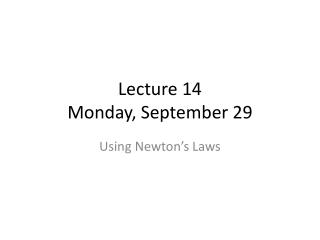DownloadDownload PresentationLecture 14 Monday, September 29

# Lecture 14 Monday, September 29

Download Presentation## Lecture 14 Monday, September 29

- - - - - - - - - - - - - - - - - - - - - - - - - - - E N D - - - - - - - - - - - - - - - - - - - - - - - - - - -
##### Presentation Transcript

1. Lecture 14Monday, September 29 Using Newton’s Laws

2. Harper’s Index • Minimum number of U.S. cities that have shortened the yellow light on traffic signals to under the legal limit: • 6

3. Today • Equilibrium FNET = 0 • Dynamics FNET ≠ 0 • Mass and Weight • Normal forces • Frictional forces • Chapter 5: 1-5

4. Equilibrium • Hence a=0 and v=constant • If v=0 and is constant, static equilibrium • If v≠0 and is constant, dynamic equilibrium

5. 300 Find the tensions, T1 and T2 in the two cords if the mass of the picture is 11.1 kg.

6. Procedure • Make free body diagram • Picture in (static) equilibrium so

7. Example Macie pulls a 40 kg rolling trunk by a strap angled at 30° from the horizontal. She pulls with a force of 40 N, and there is a 30 N rolling friction force acting on trunk. What is the trunk’s acceleration? Slide 5-17

8. Mass and Weight • Freely falling object

9. Elevators • You are standing on a scale in an elevator. • A) The elevator is moving upwards (downwards) at a steady speed. If your mass is 70 kg, what does the scale read? • B) The elevator which had been moving upwards, slows to a stop. What does the scale read during this time? • Normal force

10. Quiz • An elevator, lifted by a cable, is going up at a steady speed. • Identify the forces acting on the elevator. Make a free body diagram. • Is T greater than, equal to, or less than w? Or is there not enough information to tell? Slide 4-23

11. Friction • Static friction • Opposes motion

12. Kinetic Friction • Opposes motion when object is sliding • µk is the coefficient of kinetic friction

13. Rolling Friction • µr is the coefficient of rolling friction

14. Problem • 5:24 A 23kg child on slide inclined at 380. • What forces act on child? • Make a free body diagram • Magnitude of normal force? • If the coefficient of static friction is 0.62, will the child start to slide?

15. Problems due Today • 4: 13, 14, 17, 18, 20, 25, 29, 30, 31, 33, 35, 38, 65

16. Problems due Wednesday • Reading: 5: 6-8 Drag (skip), applying Newton’s laws • Problems: 5: CQ1, CQ3, MC24, MC26, MC30, 1, 2, 4, 7, 11, 13, 16, 19, 21